# 【ML】支持向量机（SVM）从入门到放弃再到掌握#前言

• 由线性分类任务开始
• 为何需要最大化间隔
• 怎么解决凸二次规划问题
• 对偶问题的求解
• SMO算法
• 核函数的由来和使用
• SVM与LR的异同

#SVM由线性分类开始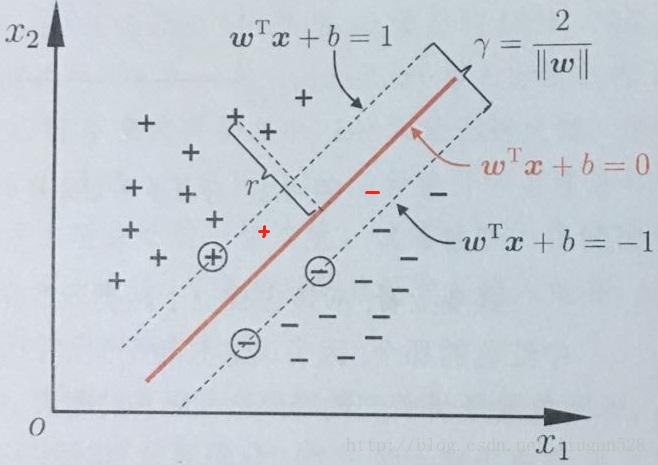$y$ =0表示反例，$y$ =300表示正例，只不过分正类的标准变为$(y-150）*f（x)>0$

$\left\{\begin{matrix}w^{T}x_{i}+b \geqslant +1, y_{i} = +1 ;& \\ w^{T}x_{i}+b \leq -1, y_{i} = -1 & \end{matrix}\right.$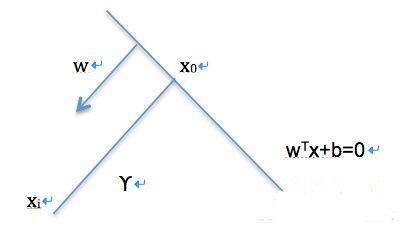$\left\{\begin{matrix}w^{T}x_{i}+b \geqslant 0, 时，对应y_{i}取正例，表示为y_{i} = +1 ;& \\ w^{T}x_{i}+b \leq 0, 时，对应y_{i}取负例，表示为 y_{i} = -1 ;& \end{matrix}\right.$

$\max{margin} \\s.t. \ y_i(w^Tx_i+b)\geqslant0 ,for \forall i = 1,...,m$

$w^{T}x_{i}+b \geqslant 0, 时，对应y_{i}取正$,
$w^{T}x_{i}+b \leq 0, 时，对应y_{i}取负$,

$\max{margin} \\s.t. \ y_i(w^Tx_i+b)\geqslant 0 ,for \forall i = 1,...,m$
margin是什么呢？

$\frac{|w^{T}x_i+b|}{\left \| w \right \|}$

$margin = 2\times _{w,b,x_i}^\textrm{min}\frac{|w^{T}x_i+b|}{\left \| w \right \|},i = 1,...,m$

$margin = 2\times _{w,b,x_i}^\textrm{min}\frac{|w^{T}x_i+b|}{\left \| w \right \|} = 2\times _{w,b,x_i,y_i}^\textrm{min}\frac{y_i(w^{T}x_i+b)}{\left \| w \right \|}, \\i = 1,...,m$

$_{w,b}^\textrm{max}\frac{1}{\left \| w \right \|}2\times _{x_i,y_i}^\textrm{min}{y_i(w^{T}x_i+b)},\\s.t.\\y_i(w^Tx_i+b)\geqslant 0,i = 1,...,m$

$\\y_i(w^Tx_i+b)\geqslant 0,i = 1,...,m$

$_{w,b}^\textrm{max}\frac{2}{\left \| w \right \|},\\s.t.\\y_i(w^Tx_i+b)\geqslant 1,i = 1,...,m$

## 接下来我们继续

$_{w,b}^\textrm{max}\frac{2}{\left \| w \right \|}$
$s.t. y_{i}( w^{T}x_{i}+b)\geq 1，i=1,2,...,m$

$_{w,b}^\textrm{min}\frac{1}{2}{\left \| w \right \|}^{2}$
$s.t. y_{i}( w^{T}x_{i}+b)\geq 1，i=1,2,...,m$

##凸优化

• 啥是凸？什么是凸优化

凸优化说的是这么一回事情，
$X\subset R^{n}$ 为一凸集，$f:X\rightarrow R$ 为一凸函数，凸优化就是要找出一点$x^{*} \in X,$使得任意$x \in X,$都满足$f(x^{*})\leq f(x)$ .
可以想象成给我一个凸函数，我要去找到最低点。当然凸优化是一个很大很厉害的领域，在这里，我们只需要知晓这个问题是这么一回事。然后，这回事要怎么样求解，就好，有兴趣的朋友可以参考凸优化的概念或者Stephen Boyd & Lieven Vandenberghe 的《Convex Optimization》。

• 为啥叫二次规划问题呢？

#对偶问题

$_{w,b}^\textrm{min}\frac{1}{2}{\left \| w \right \|}^{2}$
$s.t. y_{i}( w^{T}x_{i}+b)\geq 1，i=1,2,...,m$

• $L(w,b,\alpha )= \frac{1}{2}\left \|w\right \| ^{2} +\sum _{i=1}^{m}\alpha ^{i}(1- y_{i}( w^{T}x_{i}+b))$

$L(w,b,\alpha )= \infty$。只有约束条件均满足的时候，

$L(w,b,\alpha )$有最优值，为$L(w,b,\alpha )= \frac{1}{2}\left \|w\right \| ^{2}$

$_x^{min}f(x) \\s.t.\\m_i(x)\leqslant 0,i=1,...,m$

$\iota（x,\lambda ,\eta） = f(x) + \sum_{i=1}^{m}\lambda _im_i$

$_x^{min}{_{\lambda,\eta }^{max}}\iota (x,\lambda,\eta)$

${_{\lambda,\eta }^{max}}{_x^{min}}\iota (x,\lambda,\eta)$

## 接下来我们继续

$_{w,b}^{min}$$_{_{\alpha\geq 0}}^{max}$$L(w,b,\alpha )$

满足一定条件下，等价于（注意，这个满足一定条件，是指满足KKT条件）

$_{_{\alpha\geq 0}}^{max}$$_{w,b}^{min}$$L(w,b,\alpha )$

##KKT条件

KKT条件是一个线性规划问题能有最优解的充分和必要条件。

$minf(x)$
$s.t. h_{i}(x) = 0 , i = 1,2,...,p$
$g_{j}(x) \leq 0 , j = 1,2,...,q$
$x\in X \in R^{n}$

$h(x)$ 是等式约束。
$g(x)$ 是不等式约束。
$p,q$ 表示约束的数量。

1. $h_{i}(x^{*}) = 0 , i = 1,2,...,p$,和 $g_{j}(x^{*}) \leq 0 , j = 1,2,...,q$
2. $\bigtriangledown f(x^{*}) + \sum_{i=1}^{p}\lambda _{i}\bigtriangledown h_{i}(x^{*}) + \sum_{j=1}^{q} \mu _{k}\bigtriangledown g_{k}(x^{*}) = 0$
3. $\lambda _{i}\neq 0, \mu _{k}\geq 0, \mu _{k}g_{k}(x^{*}) = 0$

1. $L(w,b,\alpha)$$w,b$求最小

2. 再对$\alpha$ 求最大。

$w=\sum_{i=1}^{m}\alpha_{i}y_{i}x_{i},$

$0=\sum_{i=1}^{m}\alpha_{i}y_{i}.$

$_{\alpha\geq 0 }^{max}\sum_{i=1}^{m}\alpha_{i}-\frac{1} {2}\sum_{i,j=1}^{m}\alpha_{i}\alpha_{j}y_{i}y_{j}x_{i}^{T}x_{j}$

$s.t. \sum_{i=1}^{m}\alpha_{i}y_{i}.=0,$
$\alpha_{i}\geq 0 , i = 1,2,...,m$

$\left\{\begin{matrix}\alpha_{i} \geq 0 ;\\1- y_{i}( w^{T}x_{i}+b) \leq 0;\\ \alpha_{i}(1-y_{i}( w^{T}x_{i}+b))=0.\end{matrix}\right.$

##SMO算法

###############################

## 对于LR与SVM的异同我总结如下:

LR的详细介绍:https://blog.csdn.net/b285795298/article/details/88683987

## - 相同点:

• LR和SVM都是分类算法
• LR和SVM都是监督学习算法。
• LR和SVM都是判别模型
• 如果不考虑核函数，LR和SVM都是线性分类算法，也就是说他们的分类决策面都是线性的。
说明:LR也是可以用核函数的.但LR通常不采用核函数的方法.(计算量太大)

## LR和SVM不同点:

1、LR采用log损失，SVM采用合页(hinge)损失。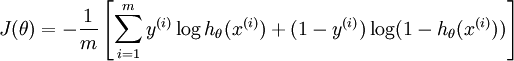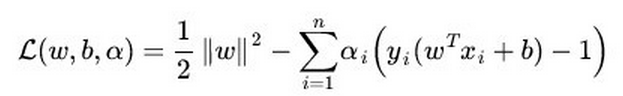​逻辑回归方法基于概率理论，假设样本为1的概率可以用sigmoid函数来表示，然后通过极大似然估计的方法估计出参数的值(基于统计的,其损失函数是人为设定的凸函数) 。支持向量机​基于几何间隔最大化原理，认为存在最大几何间隔的分类面为最优分类面.(有严格的推导)

2、LR对异常值敏感，SVM对异常值不敏感(抗燥能力,SVM要强)(https://www.jianshu.com/p/1a41a1567b87)。支持向量机只考虑局部的边界线附近的点，而逻辑回归考虑全局（远离的点对边界线的确定也起作用，虽然作用会相对小一些）。LR模型找到的那个超平面，是尽量让所有点都远离他，而SVM寻找的那个超平面，是只让最靠近中间分割线的那些点尽量远离，即只用到那些支持向量的样本。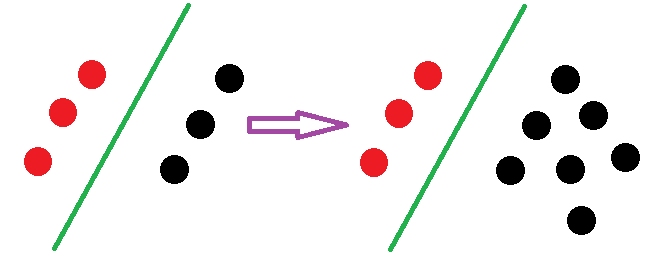逻辑回归中改变任何样本都会引起决策面的变化：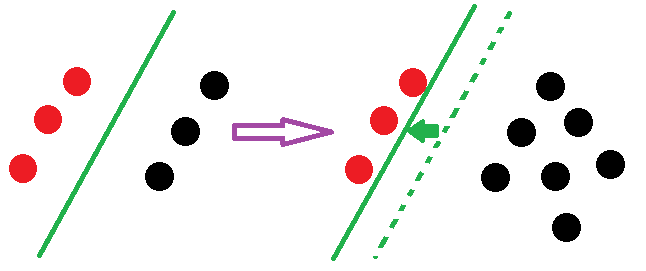LR则受所有数据点的影响，如果数据不同类别strongly unbalance，一般需要先对数据做balancing。​（引自http://www.zhihu.com/question/26768865/answer/34078149）

3、计算复杂度不同。对于海量数据，SVM的效率较低，LR效率比较高。 对于两者在feature和样本数量不同的情况下的效率问题,可以参考:https://blog.csdn.net/a244659184/article/details/81122521。该文章说明了:

4、对非线性问题的处理方式不同，LR主要靠特征构造，必须组合交叉特征，特征离散化。SVM也可以这样，还可以通过kernel(因为只有支持向量参与核计算,计算复杂度不高)。(由于可以利用核函数,。SVM则可以通过对偶求解高效处理。LR则在特征空间维度很高时，表现较差。)

5、SVM的损失函数就自带正则！！！（损失函数中的1/2||w||^2项），这就是为什么SVM是结构风险最小化算法的原因！！！而LR必须另外在损失函数上添加正则项！！！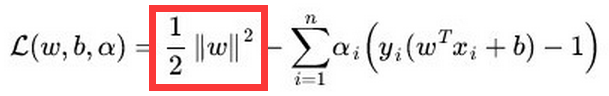1）一类是落在对应分界平面外并被正确分类的点，比如落在正分界左侧的正样本或落在负分界右侧的负样本
2）第二类是落在gap里或被错误分类的点。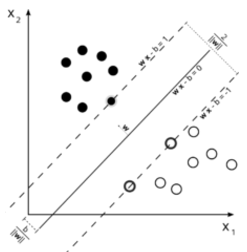normalization
，(这里的normalization是对数据的归一化,注意区分之前的LR在类别不平衡的时候做的balancing)而求解LR（without regularization）时则不需要或者结果不敏感。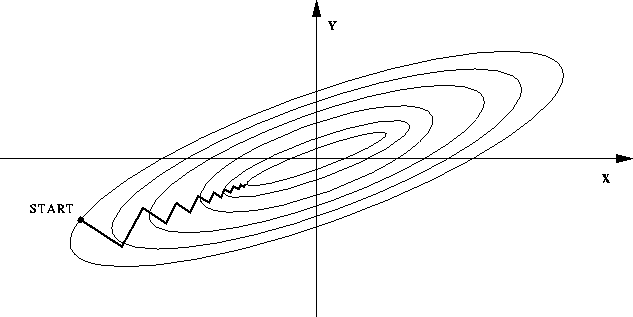（图中椭圆表示目标函数的等高线，两个坐标轴代表两个特征）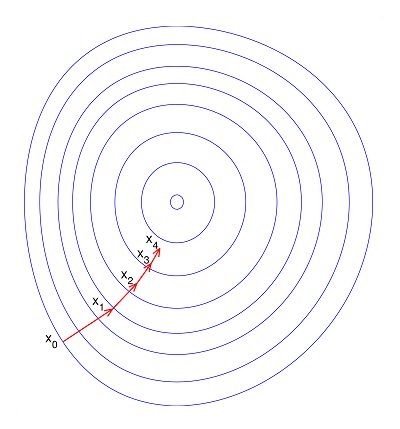每一步梯度的方向都基本指向最小值，可以大踏步地前进。（引自https://www.zhihu.com/question/37129350）

#######################################################

https://www.jianshu.com/p/1a41a1567b87
https://www.cnblogs.com/bentuwuying/p/6616761.html
https://blog.csdn.net/zwqjoy/article/details/82312783©️2019 CSDN 皮肤主题: 技术工厂 设计师: CSDN官方博客